# Bayesian Age/Period/Cohort Models in Python with PyMC

For my day job, I spend a lot of time thinking about e-commerce analytics and cohort analysis in particular. Statistical age-period-cohort (APC) models are important in many fields such as epidemiology, demography, marketing, and many more. These models also pose some interesting inferential challenges for the unwary. This post shows how to use pymc to build Bayesian APC models in Python and presents a series of increasingly sophistocated systems of priors to resolve the inferential challenges these models pose.

First we make the necessary Python imports and do some light housekeeping.

In :
%matplotlib inline
%config InlineBackend.figure_format = "retina"

In :
from warnings import filterwarnings

In :
from aesara import tensor as at
import arviz as az
from matplotlib import pyplot as plt
import numpy as np
import pandas as pd
import pymc as pm
import scipy as sp
import seaborn as sns

In :
filterwarnings("ignore", category=UserWarning, module="pymc")

In :
plt.rc("figure", figsize=(8, 6))
sns.set(color_codes=True)


For illustrative purposes, we use data from the US General Social Survey 1974-2002 in this post. This dataset is available from Vincent Arel-Bundock's excellent Rdatasets repository and is originally from the AER (Applied Econometrics with R) R package.

In :
DATA_URL = "https://vincentarelbundock.github.io/Rdatasets/csv/AER/GSS7402.csv"

In :
df = (pd.read_csv(DATA_URL, usecols=["kids", "age", "year"])
[["age", "year", "kids"]])

In :
df

Out:
age year kids
0 25 2002 0
1 30 2002 1
2 55 2002 1
3 57 2002 2
4 71 2002 2
... ... ... ...
9115 30 1998 3
9116 37 1998 2
9117 59 1998 3
9118 73 1998 2
9119 40 1998 0

9120 rows × 3 columns

### Exploratory data analysis¶

From the Rdatasets site, this data set contains

[c]ross-section data for 9120 women taken from every fourth year of the US General Social Survey between 1974 and 2002 to investigate the determinants of fertility.

The columns of this data frame are

• age: the age at which the woman was surveyed,
• year: the year the woman was surveyed, and
• kids: the number of children the woman had at the time she was surveyed.

These columns contain all the information we need to build an APC model of how the average number of children has varied as a function of the woman's age, the year, and when she was born. Obviously the age column corresponds to the "age" component of the APC model, and the year column corresponds to the "period" component of the APC model. For this data set, the "cohort" component corresponds to they year in which the woman was born, which is simply calculated as the difference between the woman's age and the current year.

In :
df["cohort"] = df["year"] - df["age"]

In :
df

Out:
age year kids cohort
0 25 2002 0 1977
1 30 2002 1 1972
2 55 2002 1 1947
3 57 2002 2 1945
4 71 2002 2 1931
... ... ... ... ...
9115 30 1998 3 1968
9116 37 1998 2 1961
9117 59 1998 3 1939
9118 73 1998 2 1925
9119 40 1998 0 1958

9120 rows × 4 columns

It is this relationship between age, year, and cohort that will make a naively parametrized APC model unidentified, causing inferential challenges.

The following plots show how the average number of childen women varies with age, period (year), and cohort.

In :
fig, axes = plt.subplots(ncols=3, nrows=2, figsize=(12, 4),
gridspec_kw={"height_ratios": (1, 5)})

axes[0, 0].sharex(axes[1, 0]);
axes[0, 1].sharex(axes[1, 1]);
axes[0, 2].sharex(axes[1, 2]);

axes[0, 0].sharey(axes[0, 1]);
axes[0, 1].sharey(axes[0, 2]);

axes[1, 0].sharey(axes[1, 1]);
axes[1, 1].sharey(axes[1, 2]);

# age

axes[0, 0].hist(
df["age"],
bins=np.arange(df["age"].min(), df["age"].max() - 1, 3),
lw=0
);

axes[0, 0].set_ylabel("Number\nof women");

(df.groupby("age")
["kids"]
.mean()
.plot(ax=axes[1, 0]));

axes[1, 0].set_xlabel("Age");
axes[1, 0].set_ylabel("Average number\nof children");

# year

axes[0, 1].hist(
df["year"],
bins=np.arange(df["year"].min(), df["year"].max() - 1),
lw=0
);

(df.groupby("year")
["kids"]
.mean()
.plot(ax=axes[1, 1]));

axes[1, 1].set_xlabel("Year");

# cohort

axes[0, 2].hist(
df["cohort"],
bins=np.arange(df["cohort"].min(), df["cohort"].max() - 1, 5),
lw=0
);

(df.groupby("cohort")
["kids"]
.mean()
.plot(ax=axes[1, 2]));

axes[1, 2].set_xlabel("Cohort (birth year)");
axes[1, 2].set_ylim(0, 4);

fig.tight_layout();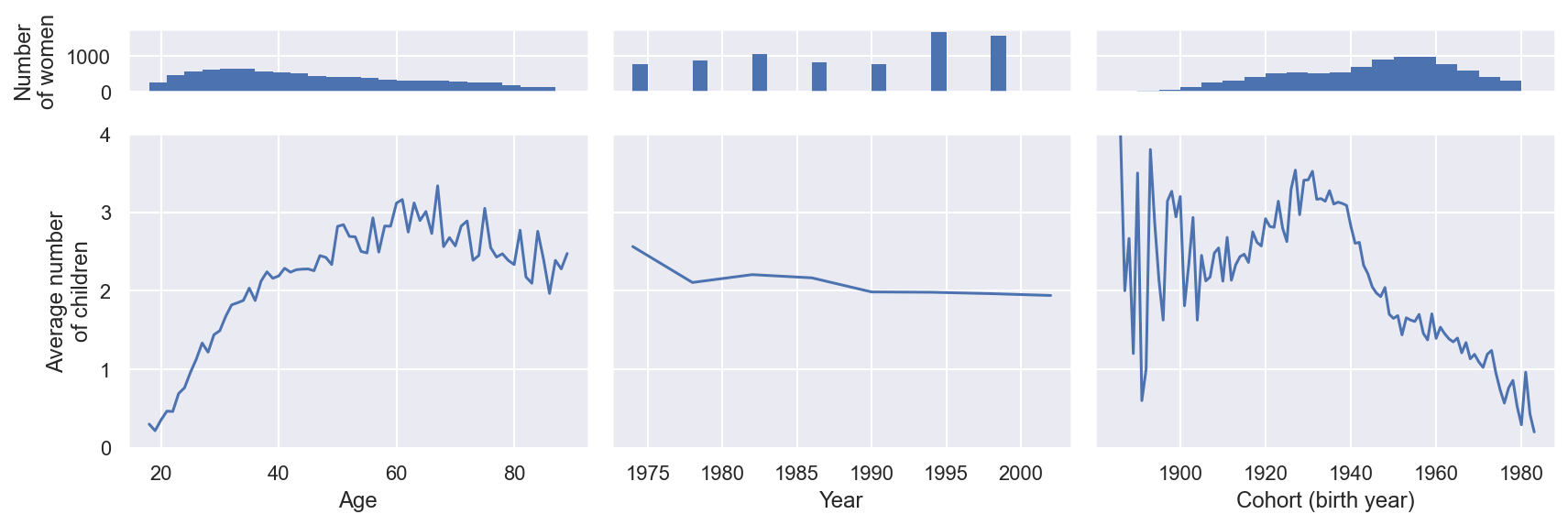The relationship with age is not surprising: throughout the twenties and thirties the average number of children increases at a relatively stable rate and then levels out from the forties onward. The most notable feature of the period relationship is that it is relatively small compared to the age and cohort relationship. The relationship with cohort is perhaps the most interesting. The year a woman is born determines when she enters the prime chilbearing years of twenty to forty, so the peaks and troughs in the cohort relationship roughly corresponding to economic and societal conditions when the women in that cohort are in prime childbearing years.

In :
GREAT_DEPRESSION = np.array([1929, 1939])
BABY_BOOM = np.array([1946, 1964])

In :
fig, ax = plt.subplots()

ax.axvspan(GREAT_DEPRESSION - 30, GREAT_DEPRESSION - 20,
color="C3", alpha=0.5, label="Great Depression");
ax.axvspan(BABY_BOOM - 30, BABY_BOOM - 20,
color="C2", alpha=0.5, label="Post-WWII Baby Boom");

(df.groupby("cohort")
["kids"]
.mean()
.plot(label="_nolegend_", ax=ax));

ax.set_xlabel("Cohort (birth year)");

ax.set_ylim(0, 4);
ax.set_ylabel("Average number of children");

ax.legend(loc="upper right", title="Chilbearing age during");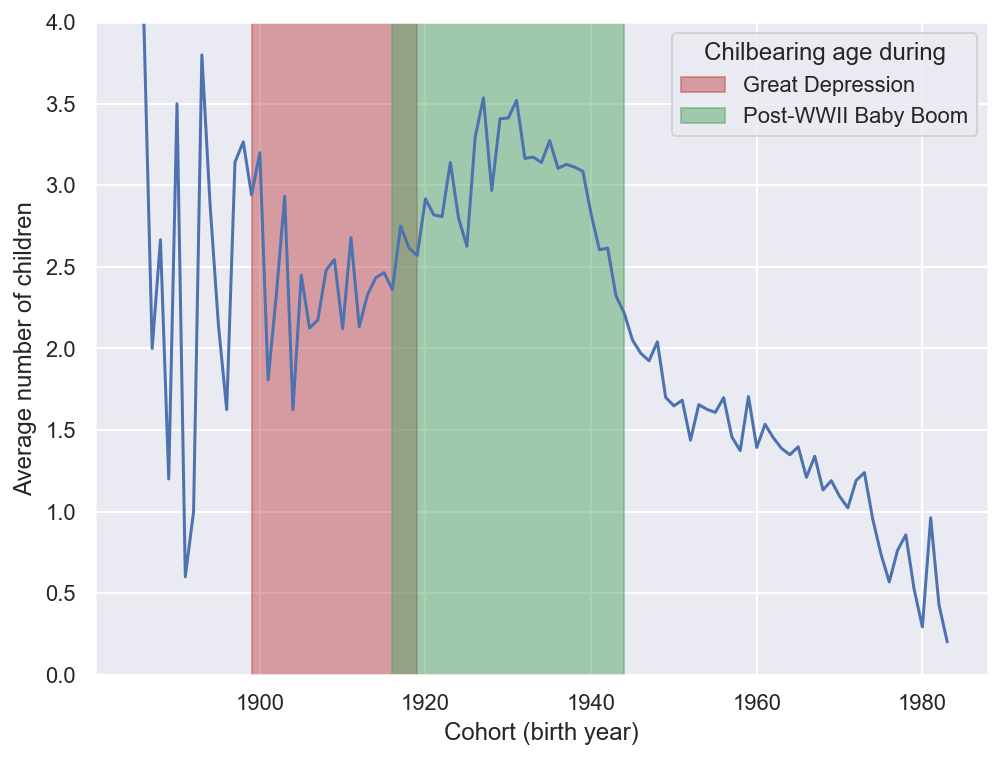Taking a closer look at the cohort relationship, we see that the first trough corresponds to women that would be having most of their children during the Great Depression, and the subsequent peak corresponds to women having children during the post-World War II baby boom.

Taken together, it is reasonable to hypothesize that biological factors related to age and socioeconomic factors related to cohort are sufficient to explain the variation in the number of children without considering period (year). The APC models we build in this post will allow us to evaluate the credibility of this hypothesis.

It is important to note at this point that I am not a demographer and even if I was, it is best not to draw deep demographic conclusions from any analysis conducted on this data set. The purpose of this post is to use this data set as a simple example to illustrate some of the subtleties involved in inference using APC models.

#### Dispersion¶

Since the number of children is discrete, we being by checking its index of dispersion in order to determine which probability distribution is best suited to model it.

In :
def index_of_dispersion(x):
return x.var() / x.mean()

In :
index_of_dispersion(df["kids"])

Out:
1.5694754102397794

We see that the number of children is overdispersed across all responses.

In :
fig, axes = plt.subplots(ncols=3, sharey=True, figsize=(12, 4))

index_of_dispersion(df.groupby("age")["kids"]).plot(label="_nolegend_", ax=axes);
axes.axhline(1, c="k", ls="--", label="Unit dispersion (Poisson)");

axes.set_xlabel("Age");
axes.set_ylabel("Index of dispersion");
axes.legend(loc="upper left");

index_of_dispersion(df.groupby("year")["kids"]).plot(ax=axes);
axes.axhline(1, c="k", ls="--");

axes.set_xlabel("Year");

index_of_dispersion(df.groupby("cohort")["kids"]).plot(ax=axes);
axes.axhline(1, c="k", ls="--");

axes.set_xlabel("Year");

fig.tight_layout();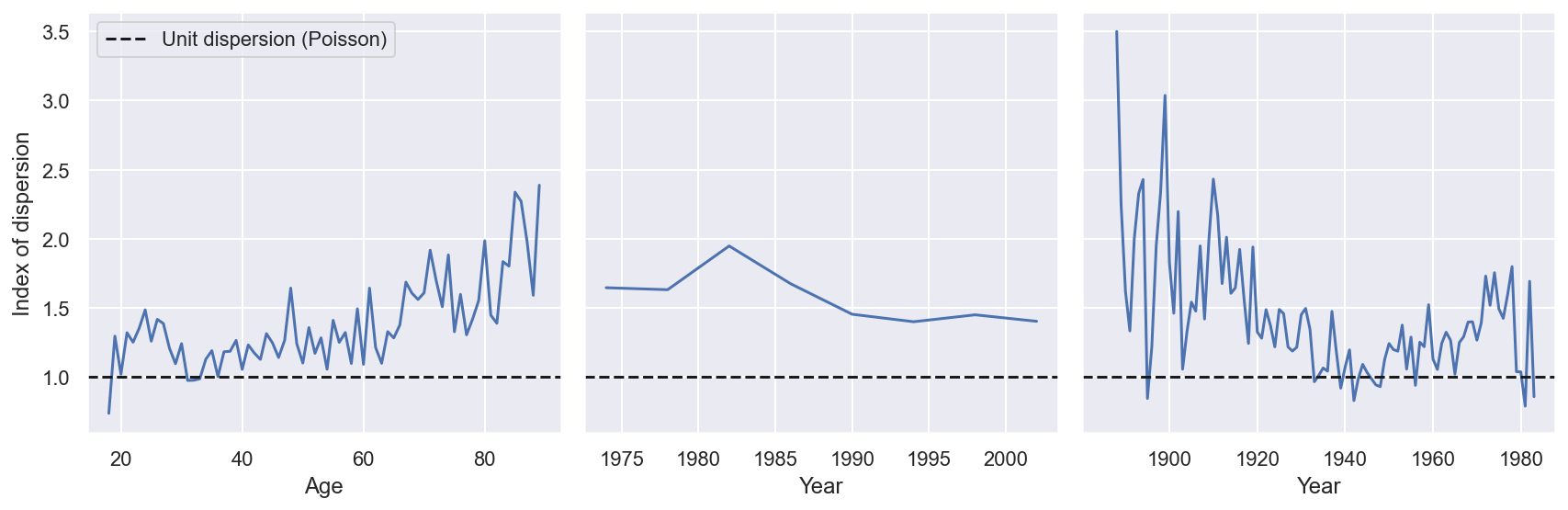The number of children continues to be overdispersed (without about the same index) across each of the APC dimensions, so we will use the overdispersed negative binomial distribution in our models.

### Modeling¶

We now start to build APC models of the number of children reported. Recall that if $X \sim \operatorname{NB}(\mu_X, \nu)$ and $Y \sim \operatorname{NB}(\mu_Y, \nu)$ then $X + Y \sim \operatorname{NB}(\mu_X + \mu_Y, \nu).$ Using this fact, we reduce the size of the observed data in our model by grouping by the age/period/cohort combination of each observation, counting the number of women with that combination, and summing their number of children.

In :
apc_df = (df.groupby(["age", "year", "cohort"])
["kids"]
.agg(("size", "sum")))

In :
apc_df

Out:
size sum
age year cohort
18 1974 1956 3 2
1978 1960 7 3
1982 1964 2 0
1986 1968 1 0
1990 1972 2 0
... ... ... ... ...
89 1986 1897 5 10
1990 1901 8 11
1994 1905 14 35
1998 1909 10 31
2002 1913 9 11

571 rows × 2 columns

In :
N = apc_df["size"].values
kids = apc_df["sum"].values


Here apc_df["size"] and N are the number of women surveyed at that specific age/period/cohort combination and apc_df["sum"] and kids are their total number of children.

For convenience we encode age, year, and cohort as ordinal factors.

In :
i, age_map = apc_df.index.get_level_values("age").factorize(sort=True)
j, year_map = apc_df.index.get_level_values("year").factorize(sort=True)
k, cohort_map = apc_df.index.get_level_values("cohort").factorize(sort=True)


#### Flat priors¶

We are now ready to build our first APC model, which will use flat priors for the age, period, and cohort effects. Throughout this post, let $i$ denote a sample's age, $j$ denote its period, and $k$ denote its cohort. All of our models will take the form

\begin{align*} \eta_{ijk} & = \eta_0 + \alpha_i + \beta_j + \gamma_k, \\ y_{ijk} & \sim \operatorname{NB}(N_{ijk} \cdot \exp(\eta_{ijk}), \nu). \end{align*}

Here $N_{ijk}$ is the number of women in the relevant age/period/cohort combination, $y_{ijk}$ is the total number of children they have, and $\nu$ controls the amount of overdispersion. Note that this is a negative binomial regression model with an offset term.

Using pymc we specify the flat prior distributions

$$\pi(\eta_0), \pi(\alpha_i), \pi(\beta_i), \pi(\gamma_i) \propto 1.$$
In :
coords = {
"age": age_map,
"year": year_map,
"cohort": cohort_map
}

In :
SEED = 1234567890 # for reproducibility

In :
with pm.Model(coords=coords, rng_seeder=SEED) as flat_model:
η0 = pm.Flat("η0")
α = pm.Flat("α", dims="age")
β = pm.Flat("β", dims="year")
γ = pm.Flat("γ", dims="cohort")


Using the prior $\nu \sim \operatorname{Half-}N(2.5^2)$, we specify $\eta_{ijk}$ and the observational likelihood as above.

In :
with flat_model:
η = η0 + α[i] + β[j] + γ[k]

ν = pm.HalfNormal("ν", 2.5)
pm.NegativeBinomial("kids", N * at.exp(η), ν, observed=kids)


We are now ready to sample from the posterior distribution of this model.

In :
CHAINS = 6

SAMPLE_KWARGS = {
"cores": CHAINS,
"random_seed": [SEED + i for i in range(CHAINS)]
}

In :
with flat_model:
flat_trace = pm.sample(**SAMPLE_KWARGS)

Auto-assigning NUTS sampler...
Multiprocess sampling (6 chains in 6 jobs)
NUTS: [η0, α, β, γ, ν]

100.00% [12000/12000 06:47<00:00 Sampling 6 chains, 3,014 divergences]
Sampling 6 chains for 1_000 tune and 1_000 draw iterations (6_000 + 6_000 draws total) took 428 seconds.
The chain contains only diverging samples. The model is probably misspecified.
The acceptance probability does not match the target. It is 0.3571, but should be close to 0.8. Try to increase the number of tuning steps.
The chain contains only diverging samples. The model is probably misspecified.
The acceptance probability does not match the target. It is 0.1821, but should be close to 0.8. Try to increase the number of tuning steps.
The acceptance probability does not match the target. It is 0.9528, but should be close to 0.8. Try to increase the number of tuning steps.
The chain reached the maximum tree depth. Increase max_treedepth, increase target_accept or reparameterize.
The chain reached the maximum tree depth. Increase max_treedepth, increase target_accept or reparameterize.
There were 14 divergences after tuning. Increase target_accept or reparameterize.
The chain reached the maximum tree depth. Increase max_treedepth, increase target_accept or reparameterize.
The chain contains only diverging samples. The model is probably misspecified.
The acceptance probability does not match the target. It is 0.2558, but should be close to 0.8. Try to increase the number of tuning steps.
The rhat statistic is larger than 1.01 for some parameters. This indicates problems during sampling. See https://arxiv.org/abs/1903.08008 for details
The effective sample size per chain is smaller than 100 for some parameters.  A higher number is needed for reliable rhat and ess computation. See https://arxiv.org/abs/1903.08008 for details


We see quite a few warnings as a result of sampling, most notably over half of the post-tuning samples resulted in divergences, and the $\hat{R}$ statistics are well above the acceptable diagnostic threshold of 1.01. For simplicty, we focus on the $\hat{R}$ statistics.

In :
flat_rhat = az.rhat(flat_trace)

In :
ax = (flat_rhat.max()
.to_array()
.to_series()
.plot(kind="barh"))
ax.axvline(1, c="k", ls="--");

ax.set_xlabel(r"$\hat{R}$");

ax.invert_yaxis();
ax.set_ylabel(None);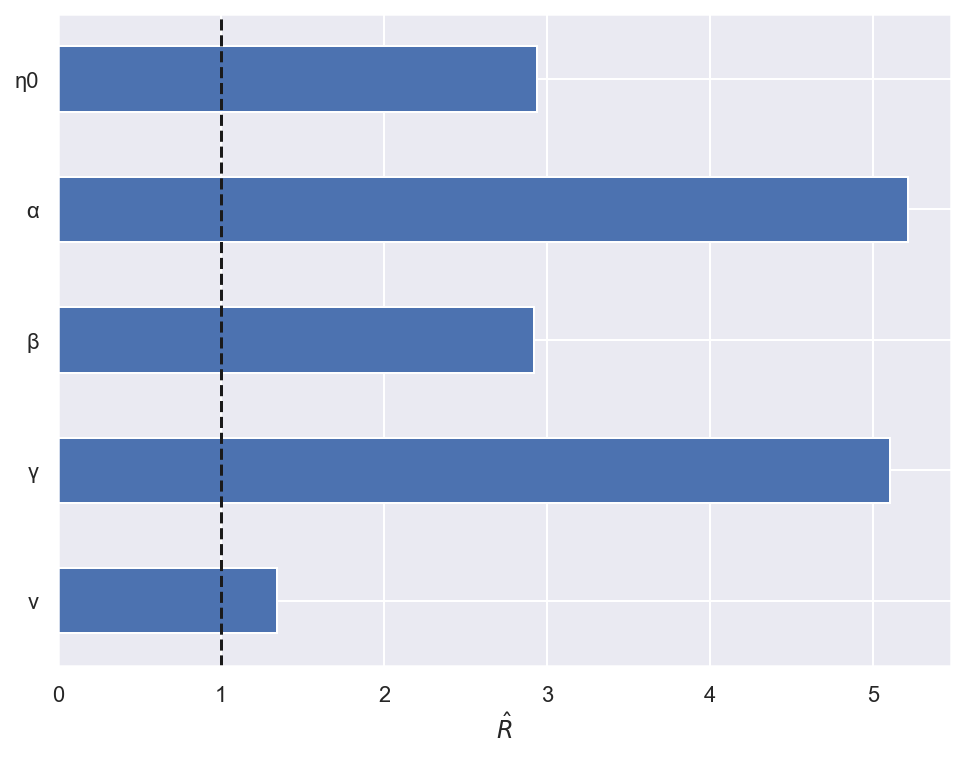We see that all of the parameters have $\hat{R}$ statistics significantly above one (two are above five) that are indicative of severe problems with inference. Focusing on the component of $\alpha$ with the highest $\hat{R}$ statistic we see that the chains are not very well mixed; they have explored completely different portions of the parameter space.

In :
α_worst = flat_rhat["α"].idxmax()

az.plot_trace(flat_trace, var_names="α", coords={"age": α_worst}, divergences=False);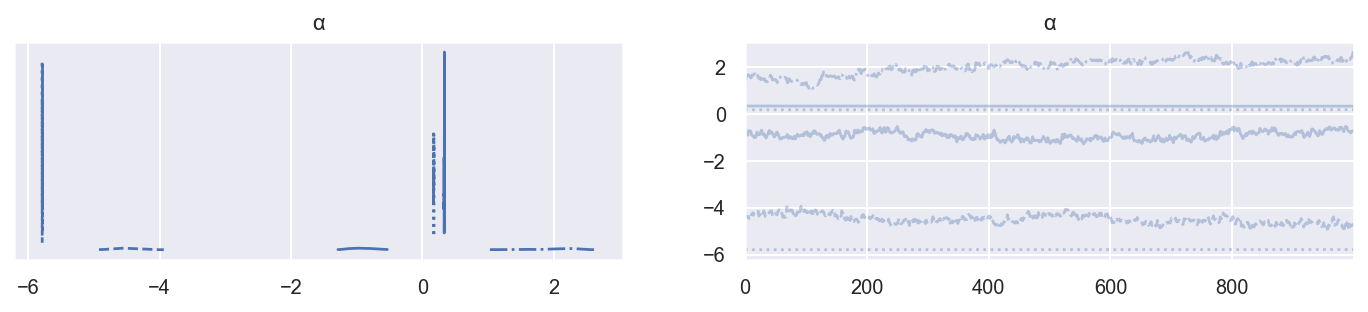To quote the folk theorem of statistical computing:

When you have computational problems, often there’s a problem with your model.

With this wisdom in mind, experience indicates that this behavior in the sampler often arises when our model is not identified, so it is time to return to our observation above that the relationship between age, year, and cohort would lead to inference problems.

#### Lack of identification¶

Briefly, a model is not identified if two different sets of parameters lead to the same (log) likelihood of the observed data. To illustrate the fact that this flat model is not identified, first we evaluate its log likelihood function when $\eta_0 = \alpha_i = \beta_j = \gamma_k = 0$ and $\nu = 1$.

In :
zero_pt = {
"η0": 0,
"α": np.zeros_like(age_map),
"β": np.zeros_like(year_map),
"γ": np.zeros_like(cohort_map),
"ν_log__": 0
}

In :
flat_logp = flat_model.compile_logp()
flat_logp(zero_pt)

Out:
array(-2731.94778354)

Since a woman's cohort (birth year) is the year she was surveyed minus her age at the time of survey, we have the following constraint between age, period and cohort.

In :
all((df["age"] - df["year"] + df["cohort"]) == 0)

Out:
True

Due to this constraint and the linearity of our model,

$$\eta_{ijk} = \eta_0 + \alpha_i + \beta_j + \gamma_k,$$

the following function produces parameters has the same likelihood as those above, given the observed data.

In :
def get_unident_pt(c=1):
return {
"η0": 0,
"α": c * age_map,
"β": -c * year_map,
"γ": c * cohort_map,
"ν_log__": 0
}

In :
flat_logp(get_unident_pt())

Out:
array(-2731.94778354)

We test this assertion for 100 random values of c in the interval $[-10, 10]$.

In :
rng = np.random.default_rng(SEED)

for c in rng.uniform(-10, 10, size=100):
np.testing.assert_allclose(flat_logp(zero_pt), flat_logp(get_unident_pt(c=c)))


This experiment confirms there are infinitely many parameter values that lead to the same likelihood.

Unidentified models such as the APC model with flat priors lead to both theoretical and practical challenges. From a theoretical perspective, we should be wary of a interpreting a model that produces infinitely many sets of parameters that are equally compatible with our data. From a computational perspective, many inference algorithms struggle with unidentified models (recall the folk theorem of statistical computing). It is important to note that these computational issues are not unique to the Bayesian approach to inference using this APC model. With flat priors, the maximum a posteriori (MAP) estimator for this model is the same as the maximum likelihood estimator (MLE).

In :
with flat_model:
flat_mle, opt_result = pm.find_MAP(return_raw=True)

100.00% [21/21 00:00<00:00 logp = -2,867, ||grad|| = 1,577.9]

In :
opt_result.success

Out:
False

We see that scipy's numerical optimizer has failed to find the MLE/MAP due to the unidentified nature of this model.

#### Normal priors¶

Regularization) is a common approach to resolving model identification problems. From a Bayesian perspective, regularization is equivalent to using non-flat priors on our parameters $\eta_0$, $\alpha_i$, $\beta_j$, and $\gamma_k$. It is reasonable to start with normally-distributed priors on these parameters. These normal priors are, in fact, equivalent to Tikhonov regularization/ridge regression.

For this model, we let $\eta_0, \alpha_i, \beta_j, \gamma_k \sim N(0, 2.5^2)$.

In :
with pm.Model(coords=coords, rng_seeder=SEED) as norm_model:
η0 = pm.Normal("η0", 0, 2.5)
α = pm.Normal("α", 0, 2.5, dims="age")
β = pm.Normal("β", 0, 2.5, dims="year")
γ = pm.Normal("γ", 0, 2.5, dims="cohort")


Since these normal priors assign the higest probability density to zero, they favor smaller parameter values if all other factors are equal, just as in Tikhonov regularization/ridge regression.

The rest of the model is defined as before.

In :
with norm_model:
η = η0 + α[i] + β[j] + γ[k]

ν = pm.HalfNormal("ν", 2.5)
pm.NegativeBinomial("kids", N * at.exp(η), ν, observed=kids)


We now sample from the posterior distribution of this model.

In :
with norm_model:
norm_trace = pm.sample(**SAMPLE_KWARGS)

Auto-assigning NUTS sampler...
Multiprocess sampling (6 chains in 6 jobs)
NUTS: [η0, α, β, γ, ν]

100.00% [12000/12000 03:05<00:00 Sampling 6 chains, 801 divergences]
Sampling 6 chains for 1_000 tune and 1_000 draw iterations (6_000 + 6_000 draws total) took 206 seconds.
The acceptance probability does not match the target. It is 0.9187, but should be close to 0.8. Try to increase the number of tuning steps.
There were 800 divergences after tuning. Increase target_accept or reparameterize.
The acceptance probability does not match the target. It is 0.6221, but should be close to 0.8. Try to increase the number of tuning steps.
There was 1 divergence after tuning. Increase target_accept or reparameterize.
The rhat statistic is larger than 1.01 for some parameters. This indicates problems during sampling. See https://arxiv.org/abs/1903.08008 for details
The effective sample size per chain is smaller than 100 for some parameters.  A higher number is needed for reliable rhat and ess computation. See https://arxiv.org/abs/1903.08008 for details


We see that overall there are fewer warnings than when we sampled from the flat model, but there are still hundreds of divergences. The $\hat{R}$ statistics are much closer to their ideal value of one, but still larger than 1.01, so we should continue to be a skeptical of the quality of these samples.

In :
norm_rhat = az.rhat(norm_trace)

In :
ax = (norm_rhat.max()
.to_array()
.to_series()
.plot(kind="barh"))
ax.axvline(1, c="k", ls="--");

ax.set_xlim(0.99, 1.08);
ax.set_xlabel(r"$\hat{R}$");

ax.invert_yaxis();
ax.set_ylabel(None);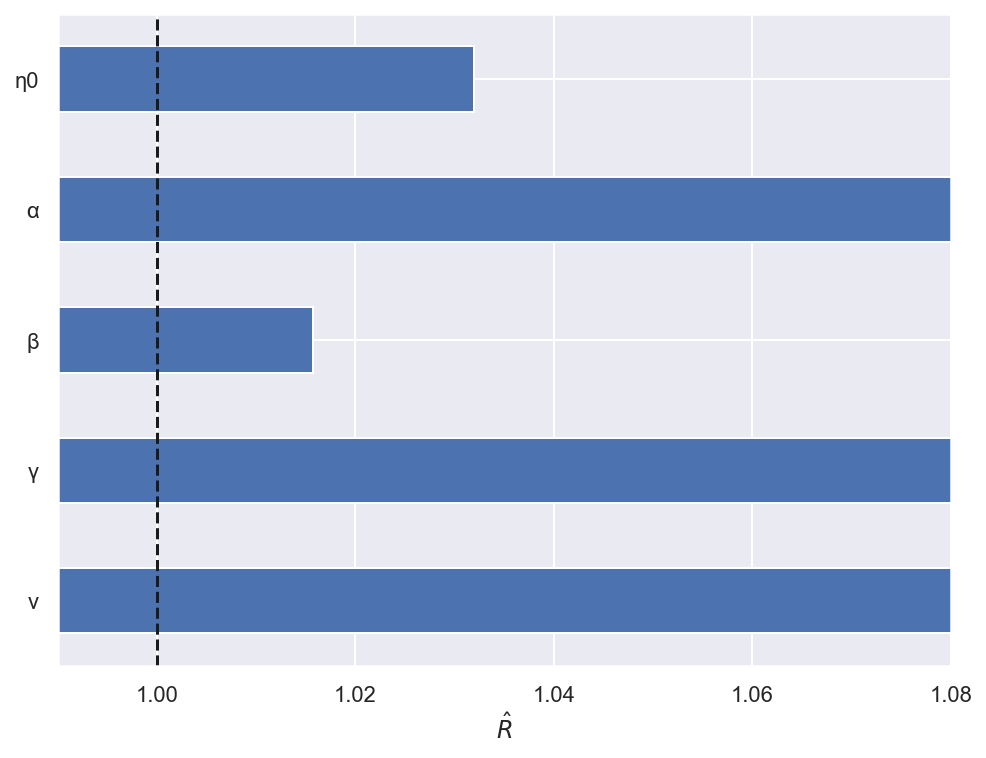To demonstrate the impact of these elevated $\hat{R}$ statistics, we visualize the samples from the $\alpha_i$ component with the highest $\hat{R}$ statistic below.

In :
α_worst = norm_rhat["α"].idxmax()

az.plot_trace(norm_trace, var_names="α", coords={"age": α_worst}, divergences=False);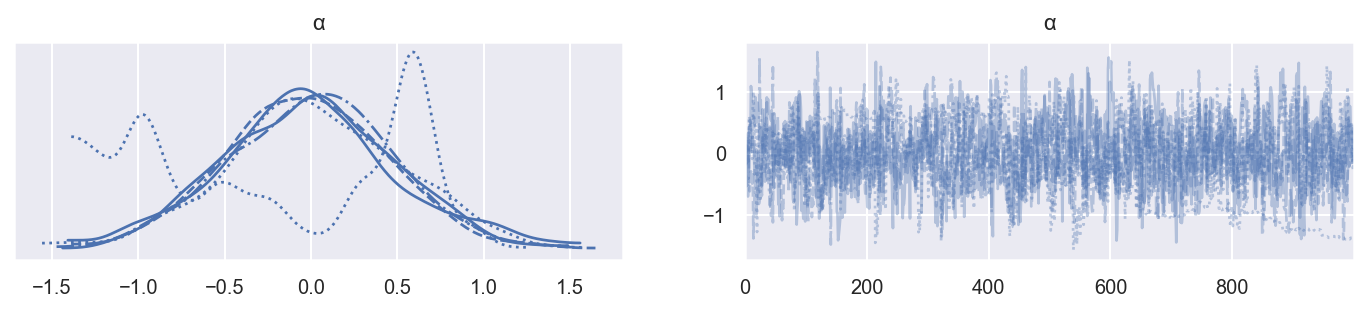In the kernel density estimates on the left, we see at least one chain that is meandering around the parameter space.

In :
fig, axes = plt.subplots(nrows=CHAINS, sharex=True, sharey=True)

for chain, ax in enumerate(axes):
(norm_trace.posterior["α"]
.sel(age=α_worst, chain=chain)
.plot(ax=ax));

ax.set_xlabel(None);

ax.set_yticks([]);
ax.set_ylabel(f"Chain {chain + 1}");

ax.set_title(None);

axes[-1].set_xlabel("Draw");

fig.suptitle("α");
fig.tight_layout();